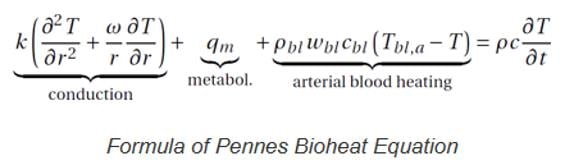## Fluids

•Siyeong
Subscriber

Hello,

I would like to implement bio-heat equation (Pennes equation) instead of solving the energy equation built in FLUENT.I found out that I can use UDS transport equation for implementing the bio-heat equation.

Could you please let me know where I should start from? or Is there any similar tutorials ?

Thank you

•DrAmine
Ansys Employee

You can start by looking into the Theory and Customization manuals in Fluent.

•Karthik R

Hello,

Just to add to abenhadj's comments, you should be able to compare the equation for UDS (refer to section 1.3.1 theory guide) with yours and appropriately add additional source terms to modify your equation. Please note that you might have to linearize your source terms.

Question: Are you solving a coupling between energy equation and this bio-heat equation? If not, you can turn off the flow (from 'Solution controls' in Fluent, and perhaps modify the energy equation for your purpose and solve a conduction problem with additional volumetric sources in your domain. Please see if you can modify the heat equation in Fluent and write it in a form similar to your bio-heat equation.

I hope this helps.

Best Regards,

Karthik